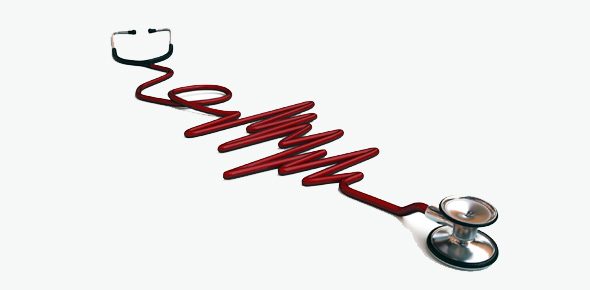# Vital Signs Quiz: Multiple Choice Questions

15 Questions | Total Attempts: 4560SettingsRelated Topics
• 1.
Vital signs includes, blood pressure, Pulse, Temp. Resp. rate & Oxygen saturation.
• A.

True

• B.

False

• 2.
Where is temperature is regulated?
• A.

Hypothalamus

• B.

Skin

• C.

Heat & Cold

• D.

By what a person wear

• 3.
What is the normal range of temperature?
• A.

36.6-37.5 c

• B.

34-36 c

• C.

34.6-36 c

• D.

35.6-38 c

• 4.
What is the normal pulse rate?
• A.

12-20

• B.

15-20

• C.

60-100

• D.

50-80

• 5.
A weak pulse is rated as
• A.

0

• B.

1+

• C.

2+

• D.

3+

• E.

4+

• 6.
A patient have a pulse rate of 102, resp. rate of 25, a B/P of 139/90 and a temp. of 103 F. As a nurse which will you assess first?
• A.

B/P of 139/90

• B.

Pulse of 102

• C.

Resp. rate of 25

• D.

Temp. of 103 F

• 7.
What is the maximum of oxygen can you give a patient with COPD?
• A.

2 L/min

• B.

10 L/min

• C.

4 L/min

• D.

6 L/min

• 8.
Blood pressure is the force against the aterial wall
• A.

True

• B.

False

• 9.
A person that is dehydrated will experience a low B/P
• A.

True

• B.

False

• 10.
What factor does not influence Resp. rate?
• A.

Age

• B.

Gender

• C.

Smoking

• D.

Medication

• E.

Pain

• 11.
COPD regulation is by hypoxemia via chemoreceptors in the brain
• A.

True

• B.

False

• 12.
Eupnea is another word for difficulty breathing
• A.

True

• B.

False

• 13.
Pulse deficit is the difference between the apical and radial rate
• A.

True

• B.

False

• 14.
A patient has a radial pulse of 65 & a apical rate of 66, What is the pulse deficit?
• A.

0

• B.

1

• C.

1+

• D.

131

• 15.
A patient with a Temp. of 104 F and a damage hypothalamus, will you give antipyretic to lower the temperature?
• A.

True

• B.

False